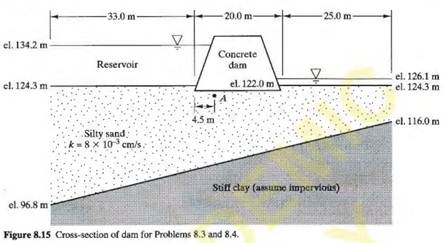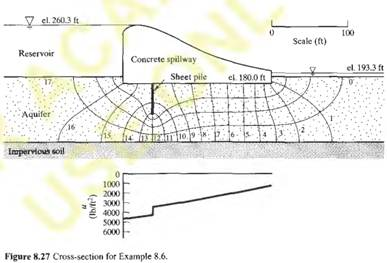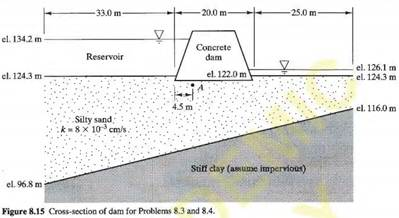### Create an Account

Already have account?

### Forgot Your Password ?

Home / Questions / Using the flow net from Problem 83 compute the uplift pressures acting on the bottom of th...

# Using the flow net from Problem 83 compute the uplift pressures acting on the bottom of the dam in Figure 8 15 and develop a plot similar to the one shown in Figure 827 Problem 83 Redraw

Using the flow net from Problem 8.3, compute the uplift pressures acting on the bottom of the dam in Figure 8. 15 and develop a plot similar to the one

shown in Figure 8.27.Problem 8.3

Redraw the cross-section in Figure 8.15 to a seal e of 1:500 (1 cm = 5 m) then draw a flow net that describes the seepage below this 150 m long concrete dam. Finally, compute the flow rate through this soil, expressed in liters per second, and the pore water pressure at Point A, which is at elevation 122.0 m.Jul 11 2020 View more View Less

#### Answer (Solved)Subscribe To Get Solution